International Journal of Energy and Power Engineering
Volume 5, Issue 4, August 2016, Pages: 141-147

Developing of New Structure of Flat Plate Solar Water Heater and Method for Calculation and Design

Zohrab Melikyan, Siranush Egnatosyan

National University of Architecture and Construction of Armenia, HVAC and Energy, Yerevan, Armenia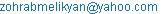(Z. Melikyan)

Zohrab Melikyan, Siranush Egnatosyan. Developing of New Structure of Flat Plate Solar Water Heater and Method for Calculation and Design. International Journal of Energy and Power Engineering. Vol. 5, No. 4, 2016, pp. 141-147. doi: 10.11648/j.ijepe.20160504.11

Received: May 1, 2016; Accepted: June 18, 2016; Published: July 6, 2016

Abstract: Single pane glazed flat solar heaters found rather wide application for domestic hot water supply. However, the practice of use shows some discrepancy between estimated and observed data: in one case the temperature of hot water does not conform to the expected pattern, in other case doubts arise about correctness of their sizes, in some cases the quantity of heated water is not enough, etc. Because of such divergence of opinions, it is becoming necessary to think about correctness of existing methods of sizing and designing of flat plate solar water heaters. This article attempts to develop a new structure and improved method for calculation and design of flat plate solar water heaters. Analysis proved relatively higher energy and cost effectiveness of the new structure of flat plate solar water heater.

Keywords: Flat, Solar, Heater, Tunnel, Similarity, Criterion, Nusselt, Prandtl

1. Introduction

The algorithm of performance of flat plate solar water heater consists of following steps

(1) Evaluation of optical losses and penetration rate of solar radiation through glazed cover.

(2) Absorption of penetrated solar radiation by absorber sheet and rising the flat plate absorber’s and consequently the hot tunnel and hotbox air temperatures. Nevertheless, as indicates H. P. Garg , usually the absorber plate temperature is unknown and is difficult to calculate or measure because it is a function of several parameters. To solve this problem the authors of this paper used a new approach allowing determining mentioned temperatures.

(3) Transferring of hot tunnel’s air heat to the water, flowing in the water metallic meander tube, laid in the hot tunnel.

(4) Storing of hot water in a tank and consume it, when there is need.

Descripted mechanism impels to use the mane principals of heat transfer and solar irradiant to developing a new, more energy efficient structure and method for calculation and design of suggested flat plate solar water heater.

2. New Structure and Operation of a Flat Plate Solar Water Heater

Fig. 1 displays the suggested structure of a flat plate solar water heater.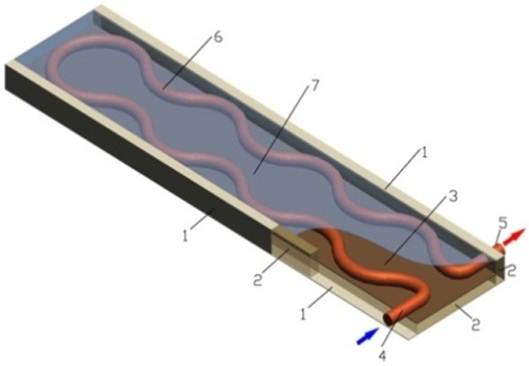Figure 1. New structure of flat plate solar water heater.

1- aluminum frame, 2- thermal insulation layer, 3- copper or aluminum absorber sheet, 4- tap water inlet tube, 5- hot water outlet tube, 6- 180o bend water meander tube, 7- glass cover

The new structure of flat plate solar water heater consists of powder coated aluminum frame (hotbox) (1) the bottom and sidewalls of which are covered by mineral wool thermal insulation layer (2). For absorbing solar rays and converting them into heat on the insulation layer (2) a copper or aluminum absorber sheet (3) and glass cover (7) are installed. On the absorber sheet a narrow air tunnel with length about l=1 m and height htun.=0.05 m is created. In the tunnel a copper, 180° bend water meander tube (6) with, exterior diameter dext.=0.024 m is laid. Through the inlet of tube (4) enters tap water and hot water exits through the outlet tube (5). Water meander tube and interior surface of the water heater are covered with dark color. The inside surface and inside air ab­sorb penetrated solar energy Qsol.in and are heated up to an appropriate conditional temperature tcond., °C. Then the solar energy Qsol.in transfers to the water, flowing in water 180° bend meander tube and heats it up to a final temperature tw.fin. The required quantity and temperature of hot water are provided by the heliosystem consisted of solar plate water heater units, arranged parallel and in series.

3. Accounting of Simultaneous Impact of Solar Radiation and Ambient Temperature on Functionality of Flat Plate Solar Water Heater

All types of solar water heaters simultaneously are sensitive to intensity of solar irradiance I, W/m2 and temperature of ambience (outside air tout). For this reason, in cold climatic conditions, the ambient temperature tout and as well the inside temperature of the hot tunnel, at nighttime and in the early morning are the same and negative. Therefore, for correct determination of absorbed solar heat Qsol.in and radiation conditional temperature tcond in tunnels, should be analyzed the daily unstable changes of solar irradiance I, W/m2, and temperature of ambience (outside air tout), which take place simultaneously. Daily changes of outside temperature of given area are shown by the following cosine harmonic function :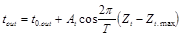(1)Figure 2. Wintertime change of outside design temperature in the course of a day for Yerevan city.

Curve 1-daily course of outside air variable temperature;

Curve 2-daily average temperature of outside air.

where:

tout - running outside temperature during a day, °C,

At - amplitude of daily temperature change (temperature fluctuation range),

Т=24 hour - daily period of oscillation of the outside temperature,

Zt - instant time of a day when given temperature occurs,

Zt.max. - instant time of the day, when outside air temperature reaches to its highest value.

The diagram of Fig. 2 represents the course of daily change of wintertime outside temperature in Yerevan city .

Based on guide of construction climatology of Armenia  the wintertime daily course of irradiance changes for Yerevan city represents the Fig. 3.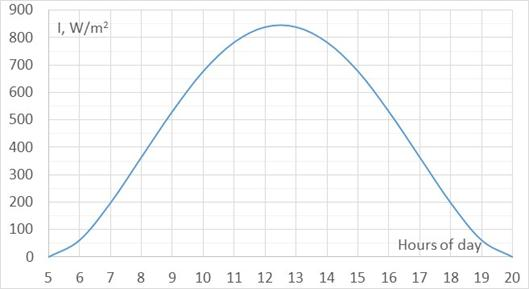Figure 3. Wintertime course of changes of irradiance I, W/m2 for Yerevan city.

From Fig. 3 it is evident that the effective sunrise takes place at 9:30 o’clock in the morning. The Fig. 2 displays that at the same 9:30 o’clock the temperature of outside air rises up to tout=-10°C, which is too low for water heating. That is why it is more probable that flat solar heater starts operating in water heating regime at 10:00 o’clock, after sunrise, when intensity of solar radiation reaches to I=655 W/m2 (see Fig. 3) and am­bient temperature becomes tout=-7°C. The mentioned combination of irradiance I=655 W/m2 and outside temperature tout=-7°C conditions thermal characteristics of flat plate solar water heater.

4. Determination of Thermal Characteristics of Flat Plate Solar Water Heater

In the early morning, before sunrise, there is not solar irradiance, penetrating into hotbox. As a result, the internal surface and ambient temperatures of hotbox are equal to outside air temperature tout that in wintertime cold climate of Armenia makes tout=-19°C. In the morning, after sunrise, solar radiation penetrates through glass cover and is absorbed by dark colored absorber sheet. The following production allows quantifying solar energy Qsol.in, W, that penetrates into narrow hot tunnel: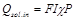(2)

where:

F-surface of glazed cover, m2,

I-solar radiation intensity, for 40° northern latitude (Armenia),

P=0.95- solar rays absorption rate by dark colored inside surface of the narrow hot tunnel .

The Fig. 3 shows that from 10:00 o’clock to 15:00 o’clock the intensity of solar radiation fluctuates and at 12:30 o’clock rises up to I=850, W/m2 and the average intensity of solar radiation at that period stays on the level I=750, W/m2.

Daily average intensity of solar radiation equals to Iav.=450 W/m2  that is typical for 40° northern latitude.

Because of partial reflection of incident solar rays from glass cover and dust level on its surface, the penetration rate of radiation through single pane glass cover makes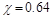. Sizes of hot tunnel of the flat plate solar water heater are as­sumed as follows: the length a=1 m, the width b=0.24 m (equal to the diameter of 180° bend of water tube) and height of narrow air tunnel hair.tun.=0.05 m, the surface of the transparent glass cover is F=0.24 m2.

According to formula (2) the following quantity of solar energy Qsol.in, W, penetrated into the hotbox is determined by the following production: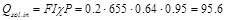W

As a result the absorber sheet and air in the tunnel acquire so called radiation conditional temperature tcond., °C, which is determined by the following formula :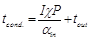(3)

where: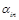- Convective heat exchange rate on internal surface of the narrow hot tunnel, W/(m2 °C).

5. Evaluation of Convective Heat Exchange Rate on Internal Surface of the Narrow Hot Tunnel

Formula (3) shows that for finding absorber sheet temperature the value of convective heat exchange rate on internal surface of hot tunnelis needed. For this purpose, the following formula from similarity theory is used [5, 6]: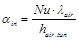(4)

where:

Nu-dimensionless Nusselt criterion (number),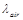-heat conductivity coefficient of air, W/(m °C),

hair.tun.- characterizing dimension of hot tunnel, m.

The value of Nu depends on the regime of air movement. In bounded spaces like hot tunnel with horizontally laid tubes, the movement of air takes place in laminar regime, in case of which the Nusselt number is evaluated by the following equation [5,6]: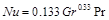(5)

where:

Gr - dimensionless criterion (number) of Grasshof.

Pr - Prandtl number. For air Pr=0.7.

The following expression presents the Grasshof number: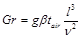(6)

where:

g – Acceleration due to gravitation force, g=9.8 m/s2,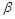=0.0032- rate of cubical expansion of air in the range of temperatures 0 to 100°C ,

l – characteristic dimension of hot tunnel, m,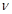- kinematic viscosity of air,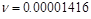m2/s.

6. Water Final Temperature in Water Tube

Assuming that whole the heat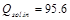W passes to the water, flowing in water meander tube the final temperature of water tw.fin can be determined by the help of the following formula: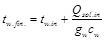(7)

where:

tw.in – initial temperature of tap water, tw.in=10°C,

gw – water flow rate in the tube, kg/s,

cw – specific heat of water cw=4180 J/(kg°C).

If daily demand of hot water makes, for instance, Gw=120 kg/day or gw=Gw / 7 h=120/7=17.1 kg/h, the final temperature of water tw.fin., according to (7), rises from initial tw.in=10°C to the following value: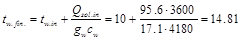°C.

The water heating up to tw.fin.=14.81°C indicates that in the hot tunnel the air temperature is higher, than tw.fin=14.81°C. This fact proves the radiation temperature tr.ab.sh of absorber sheet, on the surface of which takes place free convection: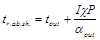(8)

where:

tout=-7°C-outside air temperature at 09:30 in the morning,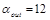W/(m2 °C)  - free heat convection rate on the surface of absorber sheet.

The equation (8) ascertains the following value of tr.ab.sh: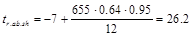°C.

Substitute β=0.0032, tair=26.2°C, l=hair.tun=0.05m and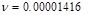in equation (6) will determine the following value for Grasshof number: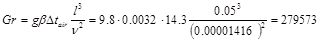Substitute the obtained value of Gr in formula (5) and making calculations will obtain the values of Nusselt and Prandtl numbers production: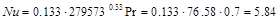Consequently, the value of convective heat transfer rate on the surface hot tunnel makes: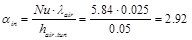W/(m2 °C)

where:

hair.tun=0.05 m – height of hot tunnel of flat plate water solar heater.

Substitute obtained values: I=655 W/m2,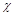=0.64, P=0.95,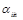=2.92 W/(m2 °C) and tout=-7°C for data in formula (3) will obtain the value of conditional temperature tcond of air on hot tunnel surface: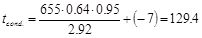°C.

7. Determination of Water Tube Diameter

The inferior diameter of water tube calculates the following formula: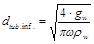(9)

where:

ω=0.015 m/s - velocity of movement of the water in tube,

ρw=1000 kg/m3 – density of water.

Substitute of above data for values in formula (9) gives the following inferior diameter of water tube: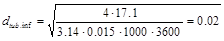m,

The exterior diameter of water tube in 3 mm is bigger, than the inferior diameter dtub.inf.=0.02 m. That is to say

dtub.ext.=0.023 m or 23 mm.

8. Determination of Required Length of Water Meander Tube

For complete transferring of the absorbed Qsol.in=95.6 W of solar energy to the water, the tube should have appropriate length ltub, m, which can be determined by the following equation: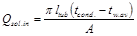(10)

where:

tw.av – average temperature of the water, in the meander tube,

A- thermal resistance to the heat transfer from air of hot tunnel to the water in the tube, m °C / W.

The average temperature of the water represents the following fraction: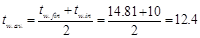°C.  (11)

The thermal resistance A to heat transfer from hot tunnel air to the water in cylindrical meander tube is calculated by the following expression :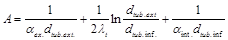(12)

where: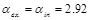W/(m2 °C) and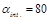W/(m2 °C) - convective heat coefficients on external and internal surfaces of water tube,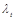=401 W/(m °C) - coefficient of heat conductivity of copper tube.

Substitution of above data for values of formula (12) and making calculation the following thermal resistance A, m °C /W to heat transfer will obtain: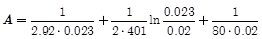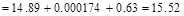m°C/W

Substitute of formals (11) and (12) in the formula (10) and making some simplifications, the following equation is obtained: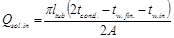(13)

For determining the required length ltub, m of water tube, the formula (13) is converted into the following equation: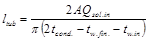(14)

For transferring completely the penetrated solar energy to the water, flowing in water tubes, the required length of water meander tube, calculated by (14) will obtain: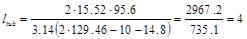m

Comparison of water meander tubes’ length with existing flat plate solar water heaters’ tubes shows that the tube of developed solar heater is much shorter. Consequently, the suggested flat plate solar water heater is simpler, compacted and cheaper.

9. Design of Heliosystem with Flat Plate Solar Water Heater Units

Considered flat plate solar water heater unit consists of hotbox’s frame with sizes a=1 m, b=0.24 m and height h.air.tun=0.05 m. In air tunnel of each unit a water heating tube with length ltub.=4 m and diameter dtub.ext.=0.023 m is laid, which provides gw=17.1 kg/h warm water with temperature tw.fin.=14.81°C. The solar units are connected each to other in form of modules and arrays. The modules are parallel installed separate units. Each of them by inlet of its water tube is connected to a tap water main pipeline, which supplies water with initial temperature tw.in.=10°C. The outlets of water tubes are connected to the warm water collector. With the help of parallel arranged units it is possible to increase the quantity of warm water of the same tw.fin.=14.81°C temperature. The number of parallel installed modules npar.mod depends on required hourly or daily quantity of hot water, which is determined by the following fraction: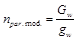(15)

where:

Gw –daily required total quantity of hot water, kg/day

gw- hourly quantity of water, heated in a flat plate unit, kg/h.

If there is necessity to get elevated temperatures of water the units should be connected each to other in series. For instance, in the second flat plate unit, connected with previous one in series, the inlet temperature of water is the outlet temperature of the previous unit tw.fin.=14.3°C. Such calculation can be realized by using the formula (7) too: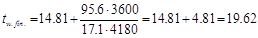°C.

where:

14.81°C– final temperature of water at the outlet of the first unit,

4.81°C – water temperature growth in each unit.

Taking into account that all the units are identic, in case of connection in series of n units the global final temperature tw.fin.gl of water will be defined by the following expression: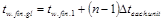(16)

where:

tw.fin.gl.- global final temperature of water at the outlet of the last unit, connected in series,

tw.fin.1 - final temperature of water at the outlet of the first unit,

n – number of all units, connected in series.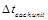- Elevation of water temperature in each next unit, connected in series.

For instance, if n=8 units are connected in series, at the outlet of the first unit the temperature of water is tw.fin=14.81°C and in each of (n-1)=7 units, connected each to other in series the temperature growth will make: 7x4.81°C =33.67°C. Therefore, the global final temperature of water at the outlet of the last 8th unit will make 14.81+33.67=48.48°C. In case of need of higher temperatures, the number of units, connected in series should be respectively increased.

If there is need for increasing of hot water quantity, can be used the heliosystem with parallel arranged units as shown in Fig. 4.

If there is need for increasing of hot water temperature, can be used the heliosystem with connections of units in series as shown in Fig. 5. The number of unites connected in series is determined by the formula as follows: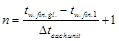(17)

For selecting the maximum number of solar water heater units that can be connected in series, should be taken into account hydraulic losses in the system 

At present, are widely used flat plate solar water heaters, the scheme of which displays the Fig. 6 .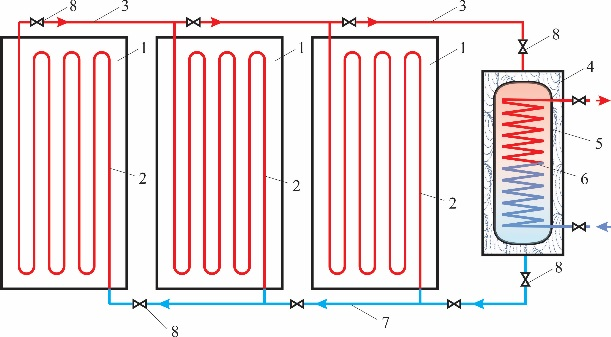Figure 4. Schematic design of heliosystem consisted of parallel-arranged flat plate solar water heating units.

1-flat plate solar water heating units, 2- water heating meander tube with length 9.06m, 3- hot water collecting pipeline, 4- thermal insulation, 5-water tank, 6- heat exchanger, 7- cold water supplying pipeline, 8-valves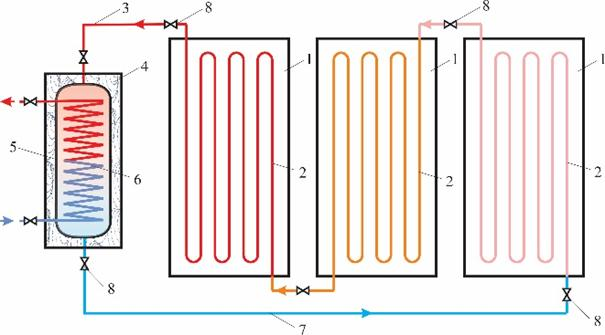Figure 5. Schematic design of heliosystem consisted of flat plate solar water heating units, connected in series.

1-flat plate solar water heating units, 2- water heating meander tubes, 3- hot water collecting pipeline, 4- water tank thermal insulation, 5-water tank, 6- heat exchanger, 7- cold water supplying pipeline, 8-valves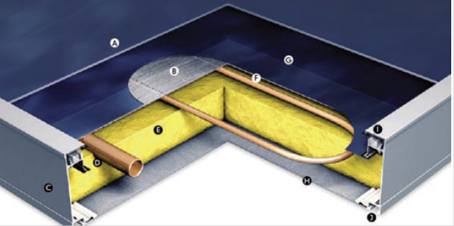Figure 6. Structure of a widely used flat plate solar water heater .

A- Glazing/ Solar Glass, B-Copper or Aluminum Absorber sheet, C-Powder Coated Aluminum Frame, D-Collector Pipe, E-Mineral Wool Insulation, F- Meander Tube, G-Selective Coating, H-Bottom Plate (Aluminum), I-Secure Glass Fixing, J-Revolving Groove for Assembly

10. Energy Efficiency and Cost Effectiveness of Flat Plate Solar Water Heater New Structure

For evaluating efficiency of suggested flat plate solar water heater, it is important to determine its initial cost. Because a sample of new flat plate solar water heater is not produced yet, there are not practically proved data on initial cost. That is why an attempt has been done to determine new solar heater initial cost by using Armenia market costs of materials and manufactured goods in accordance with main parts of flat plate solar water heater. The costs of different parts of the heater, assessed by materials and labor costs in Armenian market are the followings: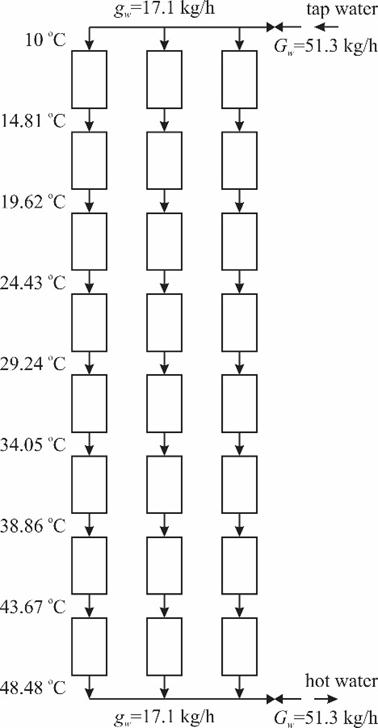Figure 7. Example of a heliosystem composed with new solar water heater units.

(1) Aluminum U- shape channel frame made of beams with total length for one unit of flat solar heater – 3 m,

cost of 1 m is 5\$/m, so the total cost of the frame - 5\$/m x 3 m=\$15,

labor cost for manufacturing of the frame \$10.

Total cost of aluminum U- shape channel frame- \$25

(2) Aluminum 1.5 mm thick and 0.237 m2 bottom plate.

cost for 1 m2 of aluminum plate-4.5\$/m2.

total cost of aluminum plate-4.5\$/m2x0.237 m2=1.1\$/m

labor cost for mounting of the bottom plate \$5.

Total cost for installing of aluminum bottom plate- \$6.1

(3) 60 mm thick and 0.2 m2 surface thermal insulation layer.

cost of 1 m2 of insulation-2\$/m2,

total cost of insulation-2\$/m2x0.2 m2=\$0.4.

labor cost for arrangement of 1 m2 of insulation – 2.5\$/m2.

Total cost of insulation of the solar heater – 2.5x0.4=\$1.0.

(4) 1.5 mm thick copper absorber sheet with surface – 0.237 m2 and weight – 9 kg.

market cost of 1 kg of cooper sheet-6.2\$,

total cost of the absorber material-6.2\$/kgx9 kg=\$55.8.

labor cost of arrangement of the absorber sheet with surface 0.237 m2-\$10.

Total cost of absorber’s installation - \$55.8+\$10=\$65.8

(5) 180° bend water meander tube with length 2.5 m:

cost of 1 m of meander tube 6\$/m.

total cost of the water meander tube 4\$/m x 2.5 m=\$10.

labor cost of arrangement of the water meander tube \$10.

Total cost of installation of the water meander tube-\$20

(6) Glass cover with surface 0.237 m2.

cost of 1 m2 of glass cover-6\$/m2. Cost of glass cover for the water heater – 6\$/m2x 0.237 m2=\$1.43

labor cost of arrangement of the glass cover-\$10.

Total cost of installation of glass cover - \$11.43

According to the above assessments, the initial (capital) cost of one unit of developed flat plate solar water heater makes: \$25+\$6+\$6.1+\$1.0+65.8+20+11.43=\$135.3.

There would be also 10% of trivial expenses, which make about \$13.53. Therefore, the total capital cost of one unit of the developed flat plate solar water heater makes \$148.8.

The structure and operation of the heliosystem shows the Fig. 7. The heliosystem consists of three rows of new flat plate solar water heater units. In each row, there are 8 units, connected in series. So, totally 24 units are used for complete heliosystem. Respectively the cost of whole the heliosystem makes 148.8x24=\$3571. The heliosystem prepares 51.3 kg/h of hot water with final temperature tw.fin.=48.48°C. Assuming that in winter and summer periods together the heliosystem performs properly in average Zd=8 h/day, the daily production of hot water makes in average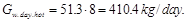As a result during a year, consisted of Zyear=295 days, the heliosystem saves energy the quantity of which can be determined by the following equation: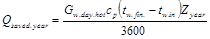(18)

Substitute above values for quantities of formula (18) will obtain Qsaved.year=5500 kWh/year of yearly saving of energy.

By replacing of gas heating boiler by suggested heliosystem, it is possible to save fuel gas during a year in the quantity, determined by the following calculation: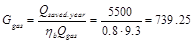m3/year

where:

ηb=0.8 - COP of gas boiler

Qgas=9.3 kWh/m3 – natural gas combustion heat.

For purchasing 739.25 m3 of gas the yearly saving of means make 739.25/1000 x \$150=110.9\$ per year. Therefore, the payback period of the proposed heliosystem makes Y=3571/110.9=32 year.

The comparison with existing flat plat solar water heaters  the payback period of suggested structure of flat plate solar heater is about twice shorter.

11. Conclusions

1. The suggested new method includes new approaches for selection of values of solar radiation and ambient temperature taking into account their simultaneous impact on the main characteristics of flat plate solar water heater.

2. The developed simplified method allows calculating all required thermo-physical and constructive characteristics needed for designing rather productive and efficient flat plate solar water heaters.

3. The detailed analysis of suggested method and obtained results prove rather efficient functionality of solar water heater in very cold climatic conditions with very low outside temperatures.

4. The suggested flat plate solar water heater produces 410 l/day of hot water and can be applied for heat supply to domestic and industrial consumers, which need hot water with temperatures up to 100°C.

5. Farther improvement of hot tunnels’ structures can increase energy efficiency of flat solar heaters.

6. Although the payback period of new flat plate solar water heater is almost twice shorter compared to existing ones, but it is steel not enough efficient. Therefore, farther simplifying of structure is needed.

References

1. H. P. Garg Treatise on Solar Energy, Volume 1: Fundamentals of Solar Energy/New York, Toronto, 1982, John Villy and Sons Ltd, p. 587, ISBN 10180 X.
2. "Constructive climatology," Constructions Codes of Armenia II-7.01-2011, Yerevan, 2013, 54 pages, Published in Armenian.
3. V. N. Bogoslovskij, "Thermal physics of constructions," Publ. house "High School", Moscow, 1982, 415 pages, Published in Russian.
4. Z. A. Melikyan, "Heating–Cooling of Buildings. Efficiency of Conventional and Renewable Technologies," LAP Lambert Academic Publishing, Germany, 2012, 344 pages ISBN- 978-3-8443-1939-2.
5. Hans Dieter Baehr, Karl Stephan, "Heat and Mass Transfer," Third revised edition, Springer-Verlag Berlin Heidelberg, 2011, 762 pages, ISBN 978-3-642-20020-5.
6. M. A. Mikheev, I. M. Mikheeva, "Heat transfer fundamentals," Second edition, Publ. House: "Energia", Moscow, 1977, 344 pages, Published in Russian.
7. "Air Properties-Temperature, density, specific heat, thermal conductivity, expansion coefficient, kinematic viscosity and Prandtl's number" http://www.engineeringtoolbox.com/air- properties-d_156.html
8. Zohrab Melikyan, Vahan Nranyan. Solar Water Heaters: Cylindrical Shell and Tube Type. Encyclopedia of Energy Engineering and Technology, Second Edition. Taylor and Francis: New York, Published online: 17 Jun 2015; 1760-1767 pp.
9. Khaled Zelzouli, Amenallah Guizani, Ramzi Sebai, Chakib Kerkeni. Solar Thermal Systems Performances versus Flat Plate Solar Collectors Connected in Series, Scientific Research, Engineering, 2012, 4, 881-893 http://dx.doi.org/10.4236 /eng.2012.412112. Published Online December 2012.
10. "Sun Maxx Titan Power Plus-SU 2 Solar Flat Plate Collectors" http://www.solarthermaltraining.org/sunmaxx-m2-solar-flat-plate-collectors.php
11. Zohrab Melikyan. Energy Efficiency and Cost Effectiveness of Solar Water Heaters. International Journal of Energy and Power Engineering. Vol. 4, No. 3, 2015, pp. 184-188. doi: 10.11648/j.ijepe.20150403.17.

 Contents 1. 2. 3. 4. 5. 6. 7. 8. 9. 10. 11.
Article ToolsAbstractPDF(836K)